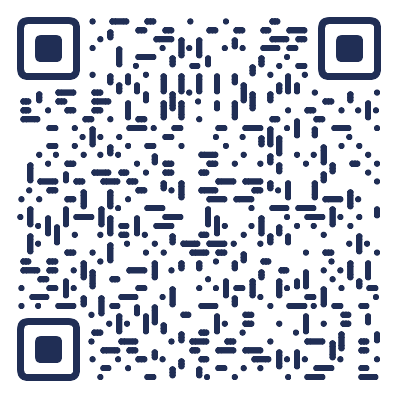double type is a strange type. And all in this type is not precision. If you want to compare two double numbers a and b are equal or not using: fabs(a-b) < threshold. And threshold may be 0.000001 or even smaller. If you want to compare two numbers a and b are equal or not correcting to 2 decimal place. you can use fabs(a-b) < 0.002, but you should not use fabs(a-b)<0.01. if a is 99.99, it will store in computer as 99.9899999, and 99.98 may be 99.97999999 or 99.98000000, so fabs(a-b) will be 0.009999, this number will be equal to 0.01. So be careful.WeChat PayAlipayPayPalBitcoin